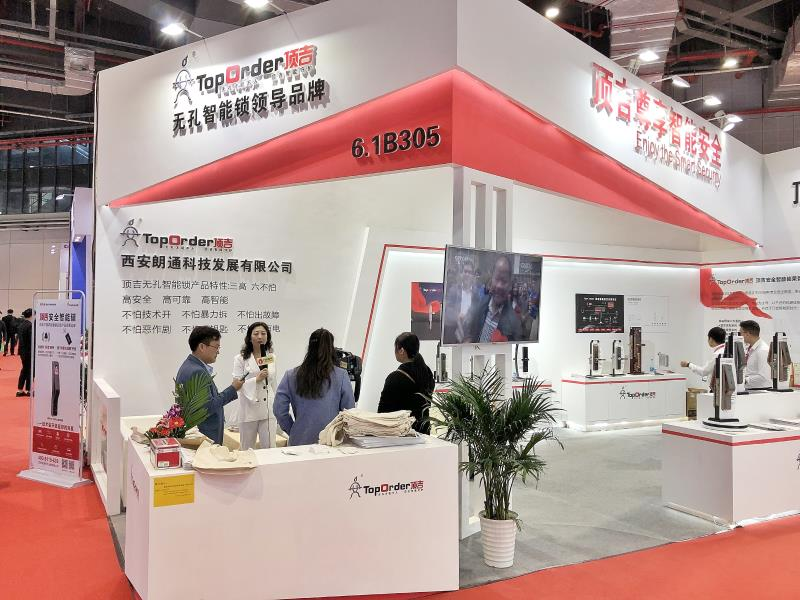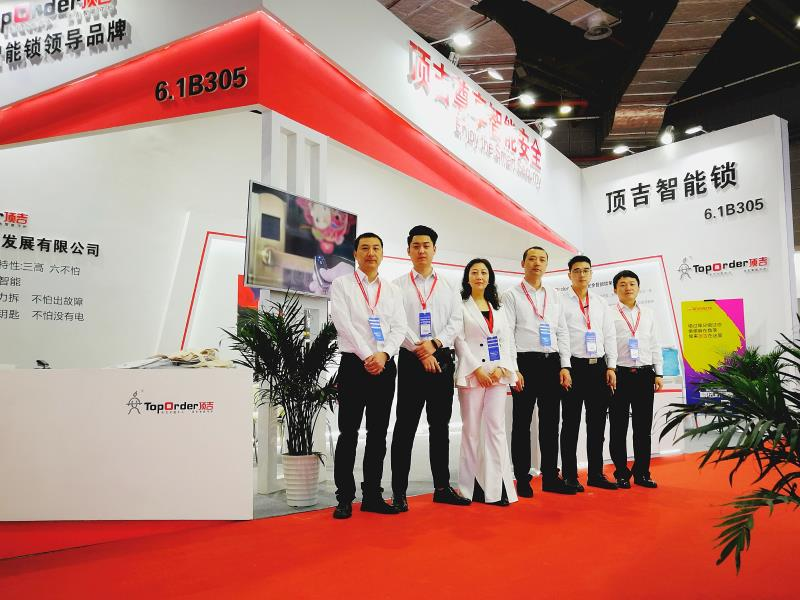|

# 品质打响品牌,西安朗通出席建博会获多家媒体关注

2019年3月26-28日，中国国际建筑贸易博览会在上海国家会展中心隆重举行。本次建博会创变新格局，开创中国同类型先进展会合作办展先河。依托两大展会无可比拟的资源优势，展会吸引了来自海内外各大客商在此经贸合作，共同演绎少有的行业盛宴。作为专业从事智能锁、家庭预警安保系统等产品的自主研发、生产、销售、服务为一体的高新技术企业，西安朗通科技发展有限公司也出席了本次建博会。13年的品牌沉淀，西安朗通科技发展有限公司将产品和市场、生活、用户接轨，秉承着“用料虽贵，必不能省;做工虽繁，必不敢简”的工匠精神，不断提升自身产品的质量，并得到了社会各界的广泛认可，此次展会现场，西安朗通就吸引了众多参展商、观众以及媒体的注意，《门锁世界》、广州慧亚文化传媒公司也在其中。专注锁具研发，缔造优质品质

《门锁世界》是一家一直致力于关注智能锁行业较新动态，解读智能锁行业趋势与未来的媒体。作为门锁行业排名前列媒体，《门锁世界》盛装参展，专注对展会盛况进行全程报道和直播。西安朗通科技发展有限公司的现场受欢迎程度让《门锁世界》感到惊奇，因此《门锁世界》采访了西安朗通的赵总。

针对《门锁世界》所提的“西安朗通的智能锁具有什么独到优势”的问题，赵总表示，西安朗通旗下的顶吉无孔智能锁，是全体西安朗通人十几年潜心研究的成果，顶吉无孔智能锁以自主知识产权、专利为主导，有着先进的门锁无孔科研优势，颠覆了传统门锁以及普通智能锁组成结构，再次刷新锁具发展新历史，可以说是开启了门锁无孔的智能新时代。

在谈及未来西安朗通会具体如何发展时，赵总表示，2018年西安朗通通过“阿里巴巴品牌出海计划”审核，成为该计划在陕西省第三家获批单位，也是中西部18省100个优选扶持企业之一。下一步，阿里巴巴将倾力扶持西安朗通，助力顶吉安全智能锁成为国际知名跨境电商品牌。

通过《门锁世界》的采访，西安朗通13年潜心研究，厚积薄发的发展历程被展现在世人面前。自2006年成立以来，西安朗通以其自身的研发能力、创新能力、实践能力为核心，以更安全、更可靠、智能、更观、更舒适的产品设计理念，秉承“无孔才能不入"的产品宗旨，一直致力于为每一位客户创领安居之家。潜心技术创新，惠及更多人群

作为家居建材行业信息资讯枢纽，广州慧亚文化传媒公司一直是市场先端产品的传播者和发现者。此次建博会现场，西安朗通旗下的先进安全智能门锁产品让广州慧亚文化传媒公司产生了浓厚兴趣。广州慧亚文化传媒公司专门采访了西安朗通的赵总，详细了解了西安朗通公司精益求精、力争上游的发展理念，以及旗下优秀的顶吉智能安全门锁产品。

此次采访中，西安朗通科技有限公司的赵总向广州慧亚文化传媒公司媒体全方位展现了该公司对门锁技术孜孜不倦、精益求精的研发精神和客户至上的专业服务精神，让世人看到了西安朗通致力于将顶吉智能家居门锁的品牌影响力推广至全国，甚至走出国门的决心。

面对国外先进发展的智能型门锁技术，赵总表示，西安朗通迎难而上，历经十余年投巨资研发，突破传统的锁业技术困境最终研发出顶吉安全智能锁，为的就是希望中国智能门锁技术能够追赶上世界的脚步，让中国创造力、创新力走向世界先进前列。随着未来科技不断地发展，智能门锁家居不再仅服务于少数高端消费人群，将逐步走向中低端人群，对此西安朗通的赵总也对自家公司的产品的发展趋势作出了介绍。其旗下顶吉智能门锁产品针对不同的消费群体推出了不同类型的门锁，力求满足需求不同的个性化消费者。公司的服务业务也已经迈进全国绝大部分省市，有着200多家终端专卖店，西安朗通力求较大程度的为不同的客户带来满意的消费体验。

通过采访，西安朗通对智能化门锁技术创新的坚持，对消费者认真的服务态度为更多的人所知道，通过技术的不断创新发展推陈出新，西安朗通立志将产品服务惠及更多消费者，为世界带来更好的智能门锁产品。

乐于追求先进，成就梦想之家

本次建博会上，西安朗通还给观众带来两款出色的新品智能门锁，一展品牌风采。顶吉T200Q全自动报警系列智能门锁和顶吉T200R型全自动人脸识别系列智能门锁就是这次西安朗通在展会上精心推出的两款产品。顶吉T200Q全自动报警系列智能门锁创新采用活体指纹、虚位密码、卡片、钥匙以及组合解锁的五种智能安全开锁方式，让用户隐私安全升级，家居安全便利。另一款顶吉T200R型全自动人脸识别系列智能门锁同样毫不逊色，创新采用红外三维立体识别技术，3D立体面部识别，给用户更安全的守护。无论是打造顶吉智能锁，还是研发其他产品，西安朗通都竭尽匠心，以自主创新为己任，以研发出更安全、更智能、更便捷、更舒适的产品为目标，以顺应市场、对接市场、领航市场为根本，用品质打响品牌，以精工成就精彩。随着其研发能力的不断增强，目前西安朗通的产品已经覆盖全国各地并且应用于相关重点安保部门，得到了社会各界的广泛认可。未来，西安朗通作为业界知名，用户信赖的优秀锁具品牌，一定会将锁具带向更智能的新时代。

`声明：本文由入驻焦点开放平台的作者撰写，除焦点官方账号外，观点仅代表作者本人，不代表焦点立场错误信息举报电话： 400-099-0099，邮箱：jubao@vip.sohu.com，或点此进行意见反馈，或点此进行举报投诉。`A B C D E F G H J K L M N P Q R S T W X Y Z
A - B - C - D - E
• A
• 鞍山
• 安庆
• 安阳
• 安顺
• 安康
• 澳门
• B
• 北京
• 保定
• 包头
• 巴彦淖尔
• 本溪
• 蚌埠
• 亳州
• 滨州
• 北海
• 百色
• 巴中
• 毕节
• 保山
• 宝鸡
• 白银
• 巴州
• C
• 承德
• 沧州
• 长治
• 赤峰
• 朝阳
• 长春
• 常州
• 滁州
• 池州
• 长沙
• 常德
• 郴州
• 潮州
• 崇左
• 重庆
• 成都
• 楚雄
• 昌都
• 慈溪
• 常熟
• D
• 大同
• 大连
• 丹东
• 大庆
• 东营
• 德州
• 东莞
• 德阳
• 达州
• 大理
• 德宏
• 定西
• 儋州
• 东平
• E
• 鄂尔多斯
• 鄂州
• 恩施
F - G - H - I - J
• F
• 抚顺
• 阜新
• 阜阳
• 福州
• 抚州
• 佛山
• 防城港
• G
• 赣州
• 广州
• 桂林
• 贵港
• 广元
• 广安
• 贵阳
• 固原
• H
• 邯郸
• 衡水
• 呼和浩特
• 呼伦贝尔
• 葫芦岛
• 哈尔滨
• 黑河
• 淮安
• 杭州
• 湖州
• 合肥
• 淮南
• 淮北
• 黄山
• 菏泽
• 鹤壁
• 黄石
• 黄冈
• 衡阳
• 怀化
• 惠州
• 河源
• 贺州
• 河池
• 海口
• 红河
• 汉中
• 海东
• 怀来
• I
• J
• 晋中
• 锦州
• 吉林
• 鸡西
• 佳木斯
• 嘉兴
• 金华
• 景德镇
• 九江
• 吉安
• 济南
• 济宁
• 焦作
• 荆门
• 荆州
• 江门
• 揭阳
• 金昌
• 酒泉
• 嘉峪关
K - L - M - N - P
• K
• 开封
• 昆明
• 昆山
• L
• 廊坊
• 临汾
• 辽阳
• 连云港
• 丽水
• 六安
• 龙岩
• 莱芜
• 临沂
• 聊城
• 洛阳
• 漯河
• 娄底
• 柳州
• 来宾
• 泸州
• 乐山
• 六盘水
• 丽江
• 临沧
• 拉萨
• 林芝
• 兰州
• 陇南
• M
• 牡丹江
• 马鞍山
• 茂名
• 梅州
• 绵阳
• 眉山
• N
• 南京
• 南通
• 宁波
• 南平
• 宁德
• 南昌
• 南阳
• 南宁
• 内江
• 南充
• P
• 盘锦
• 莆田
• 平顶山
• 濮阳
• 攀枝花
• 普洱
• 平凉
Q - R - S - T - W
• Q
• 秦皇岛
• 齐齐哈尔
• 衢州
• 泉州
• 青岛
• 清远
• 钦州
• 黔南
• 曲靖
• 庆阳
• R
• 日照
• 日喀则
• S
• 石家庄
• 沈阳
• 双鸭山
• 绥化
• 上海
• 苏州
• 宿迁
• 绍兴
• 宿州
• 三明
• 上饶
• 三门峡
• 商丘
• 十堰
• 随州
• 邵阳
• 韶关
• 深圳
• 汕头
• 汕尾
• 三亚
• 三沙
• 遂宁
• 山南
• 商洛
• 石嘴山
• T
• 天津
• 唐山
• 太原
• 通辽
• 铁岭
• 泰州
• 台州
• 铜陵
• 泰安
• 铜仁
• 铜川
• 天水
• 天门
• W
• 乌海
• 乌兰察布
• 无锡
• 温州
• 芜湖
• 潍坊
• 威海
• 武汉
• 梧州
• 渭南
• 武威
• 吴忠
• 乌鲁木齐
X - Y - Z
• X
• 邢台
• 徐州
• 宣城
• 厦门
• 新乡
• 许昌
• 信阳
• 襄阳
• 孝感
• 咸宁
• 湘潭
• 湘西
• 西双版纳
• 西安
• 咸阳
• 西宁
• 仙桃
• 西昌
• Y
• 阳泉
• 运城
• 营口
• 盐城
• 扬州
• 鹰潭
• 宜春
• 烟台
• 宜昌
• 岳阳
• 益阳
• 永州
• 阳江
• 云浮
• 玉林
• 宜宾
• 雅安
• 玉溪
• 延安
• 榆林
• 银川
• Z
• 张家口
• 镇江
• 舟山
• 漳州
• 淄博
• 枣庄
• 郑州
• 周口
• 驻马店
• 株洲
• 张家界
• 珠海
• 湛江
• 肇庆
• 中山
• 自贡
• 资阳
• 遵义
• 昭通
• 张掖
• 中卫

1室1厅1厨1卫1阳台

1
2
3
4
5

0
1
2

1

1

0
1
2
3报名成功，资料已提交审核A B C D E F G H J K L M N P Q R S T W X Y Z
A - B - C - D - E
• A
• 鞍山
• 安庆
• 安阳
• 安顺
• 安康
• 澳门
• B
• 北京
• 保定
• 包头
• 巴彦淖尔
• 本溪
• 蚌埠
• 亳州
• 滨州
• 北海
• 百色
• 巴中
• 毕节
• 保山
• 宝鸡
• 白银
• 巴州
• C
• 承德
• 沧州
• 长治
• 赤峰
• 朝阳
• 长春
• 常州
• 滁州
• 池州
• 长沙
• 常德
• 郴州
• 潮州
• 崇左
• 重庆
• 成都
• 楚雄
• 昌都
• 慈溪
• 常熟
• D
• 大同
• 大连
• 丹东
• 大庆
• 东营
• 德州
• 东莞
• 德阳
• 达州
• 大理
• 德宏
• 定西
• 儋州
• 东平
• E
• 鄂尔多斯
• 鄂州
• 恩施
F - G - H - I - J
• F
• 抚顺
• 阜新
• 阜阳
• 福州
• 抚州
• 佛山
• 防城港
• G
• 赣州
• 广州
• 桂林
• 贵港
• 广元
• 广安
• 贵阳
• 固原
• H
• 邯郸
• 衡水
• 呼和浩特
• 呼伦贝尔
• 葫芦岛
• 哈尔滨
• 黑河
• 淮安
• 杭州
• 湖州
• 合肥
• 淮南
• 淮北
• 黄山
• 菏泽
• 鹤壁
• 黄石
• 黄冈
• 衡阳
• 怀化
• 惠州
• 河源
• 贺州
• 河池
• 海口
• 红河
• 汉中
• 海东
• 怀来
• I
• J
• 晋中
• 锦州
• 吉林
• 鸡西
• 佳木斯
• 嘉兴
• 金华
• 景德镇
• 九江
• 吉安
• 济南
• 济宁
• 焦作
• 荆门
• 荆州
• 江门
• 揭阳
• 金昌
• 酒泉
• 嘉峪关
K - L - M - N - P
• K
• 开封
• 昆明
• 昆山
• L
• 廊坊
• 临汾
• 辽阳
• 连云港
• 丽水
• 六安
• 龙岩
• 莱芜
• 临沂
• 聊城
• 洛阳
• 漯河
• 娄底
• 柳州
• 来宾
• 泸州
• 乐山
• 六盘水
• 丽江
• 临沧
• 拉萨
• 林芝
• 兰州
• 陇南
• M
• 牡丹江
• 马鞍山
• 茂名
• 梅州
• 绵阳
• 眉山
• N
• 南京
• 南通
• 宁波
• 南平
• 宁德
• 南昌
• 南阳
• 南宁
• 内江
• 南充
• P
• 盘锦
• 莆田
• 平顶山
• 濮阳
• 攀枝花
• 普洱
• 平凉
Q - R - S - T - W
• Q
• 秦皇岛
• 齐齐哈尔
• 衢州
• 泉州
• 青岛
• 清远
• 钦州
• 黔南
• 曲靖
• 庆阳
• R
• 日照
• 日喀则
• S
• 石家庄
• 沈阳
• 双鸭山
• 绥化
• 上海
• 苏州
• 宿迁
• 绍兴
• 宿州
• 三明
• 上饶
• 三门峡
• 商丘
• 十堰
• 随州
• 邵阳
• 韶关
• 深圳
• 汕头
• 汕尾
• 三亚
• 三沙
• 遂宁
• 山南
• 商洛
• 石嘴山
• T
• 天津
• 唐山
• 太原
• 通辽
• 铁岭
• 泰州
• 台州
• 铜陵
• 泰安
• 铜仁
• 铜川
• 天水
• 天门
• W
• 乌海
• 乌兰察布
• 无锡
• 温州
• 芜湖
• 潍坊
• 威海
• 武汉
• 梧州
• 渭南
• 武威
• 吴忠
• 乌鲁木齐
X - Y - Z
• X
• 邢台
• 徐州
• 宣城
• 厦门
• 新乡
• 许昌
• 信阳
• 襄阳
• 孝感
• 咸宁
• 湘潭
• 湘西
• 西双版纳
• 西安
• 咸阳
• 西宁
• 仙桃
• 西昌
• Y
• 阳泉
• 运城
• 营口
• 盐城
• 扬州
• 鹰潭
• 宜春
• 烟台
• 宜昌
• 岳阳
• 益阳
• 永州
• 阳江
• 云浮
• 玉林
• 宜宾
• 雅安
• 玉溪
• 延安
• 榆林
• 银川
• Z
• 张家口
• 镇江
• 舟山
• 漳州
• 淄博
• 枣庄
• 郑州
• 周口
• 驻马店
• 株洲
• 张家界
• 珠海
• 湛江
• 肇庆
• 中山
• 自贡
• 资阳
• 遵义
• 昭通
• 张掖
• 中卫• 手机• 分享
• 设计
免费设计
• 计算器
装修计算器
• 联系
• 置顶
返回顶部